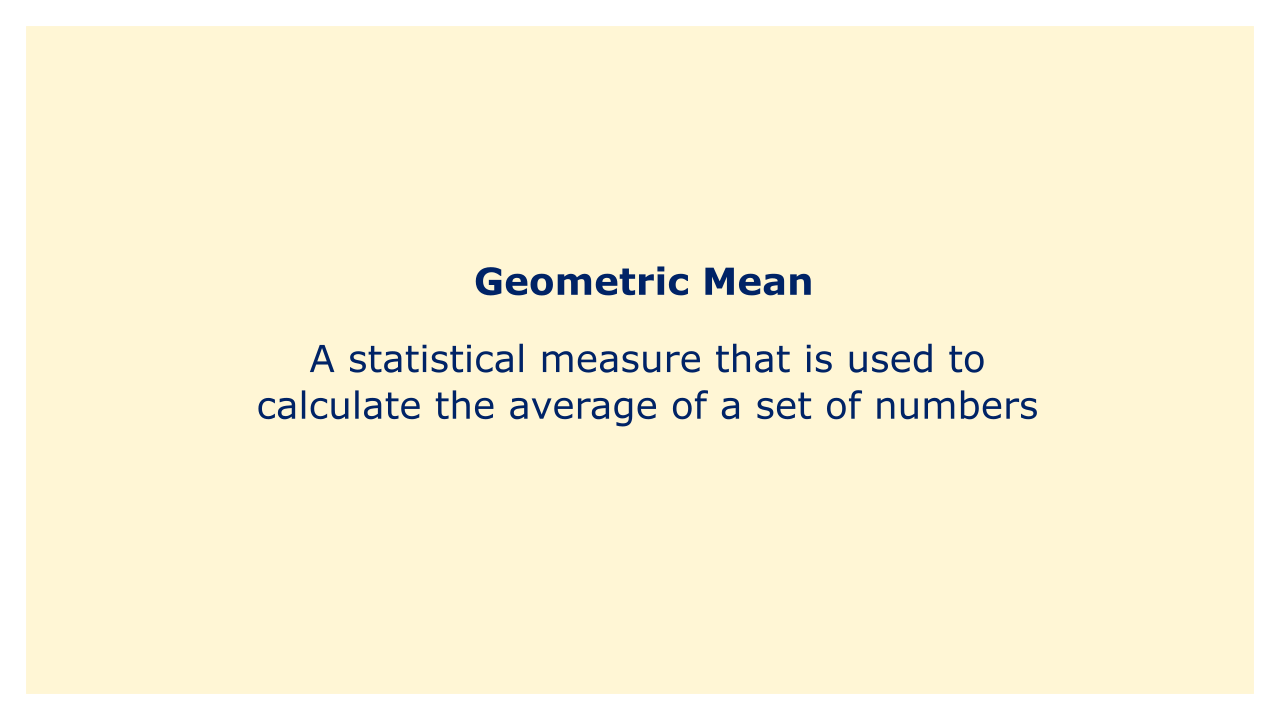# Geometric MeanImage: Moneybestpal.com

### A geometric mean is a statistical measure that is used to calculate the average of a set of numbers. The geometric mean is computed by multiplying all the numbers together and getting the nth root of the product, where n is the total number of values, as opposed to the arithmetic mean, which is just the sum of the numbers divided by the entire number of values.

The geometric mean is frequently used to determine growth rates, such as the average annual return on a portfolio of investments where returns are compounded. This is so that, in contrast to the arithmetic mean, the geometric mean accounts for the compounding effect of returns over time.

Consider a scenario where your investment portfolio generates 10% in year one, 20% in year two, and -5% in year three. By adding together these returns and dividing by three, you can determine the arithmetic mean, which yields an average annual return of 8.33%. Nevertheless, to determine the geometric mean, you would multiply the returns collectively, take the cube root (because there are three values), and deduct 1 from the result, giving you an average annual return of 8.55%.

Calculating an investment's compound annual growth rate (CAGR), a statistic of the rate of return necessary to transform an initial investment into a specific end value over a predetermined amount of time, is a common task in finance that frequently uses the geometric mean.
Tags